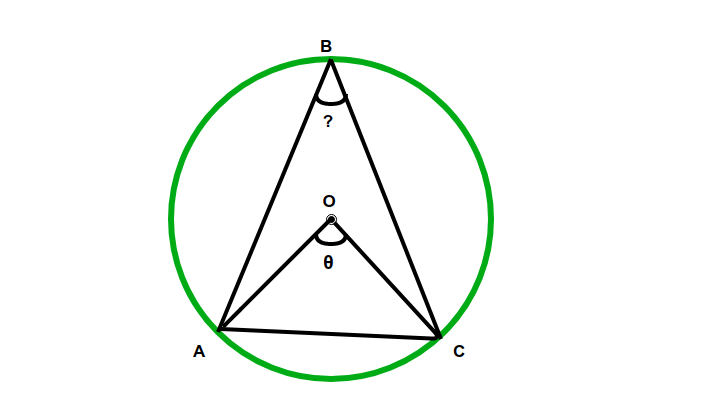# Program to calculate angle on circumference subtended by the chord when the central angle subtended by the chord is given

Given a circle having a chord and an angle subtended by chord on center of the circle. The task here is to find the measure of the angle subtended by given chord on the circumference.Examples:

Input:= 90
Output: ABC = 45.00 degrees

Input:= 65
Output: ABC = 32.50 degrees


## Recommended: Please try your approach on {IDE} first, before moving on to the solution.

Approach:

• Let AC be an chord of a circle with centre O, and let C be any point on the circumference anywhere.
• Let, angle AOC(on center) is the given.
• So angle should be on the circumference,
angle ABC = angle AOC/2

An angle at the circumference of a circle is the half angle at the centre subtended by the same chord.

Below is the implementation of the above approach:

## C++

 // C++ Program to calculate angle   // on the circumference subtended   // by the chord when the central angle  // subtended by the chord is given  #include  using namespace std;     float angleOncirCumference(float z)  {      return (z / 2);  }     // Driver code  int main()   {         // Angle on center      float angle = 65;         float z = angleOncirCumference(angle);         cout << "The angle is " << (z) << " degrees";         return 0;  }     // This code is contributed by jit_t

## Java

 // Java Program to calculate angle on the circumference  // subtended by the chord when the central angle  // subtended by the chord is given     class GFG {         static float angleOncirCumference(float z)      {          return (z / 2);      }         // Driver code      public static void main(String[] args)      {             // Angle on center          float angle = 65;             float z = angleOncirCumference(angle);             System.out.println("The angle is "                            + z + " degrees");      }  }

## Python3

 # Python3 Program to calculate angle   # on the circumference subtended   # by the chord when the central angle  # subtended by the chord is given  def angleOncirCumference(z):         return (z / 2);     # Driver code     # Angle on center  angle = 65;     z = angleOncirCumference(angle);     print("The angle is", (z), "degrees");     # This code is contributed by Rajput-Ji

## C#

 // C# Program to calculate angle on the circumference  // subtended by the chord when the central angle  // subtended by the chord is given   using System;         public class GFG   {         static float angleOncirCumference(float z)      {          return (z / 2);      }         // Driver code      public static void Main(String[] args)      {             // Angle on center          float angle = 65;             float z = angleOncirCumference(angle);             Console.WriteLine("The angle is "                         + z + " degrees");      }  }     // This code is contributed by Rajput-Ji

Output:

The angle is 32.5 degrees


Attention reader! Don’t stop learning now. Get hold of all the important DSA concepts with the DSA Self Paced Course at a student-friendly price and become industry ready.

My Personal Notes arrow_drop_upJust another competitive programmer and hard worker

If you like GeeksforGeeks and would like to contribute, you can also write an article using contribute.geeksforgeeks.org or mail your article to contribute@geeksforgeeks.org. See your article appearing on the GeeksforGeeks main page and help other Geeks.

Please Improve this article if you find anything incorrect by clicking on the "Improve Article" button below.

Improved By : Rajput-Ji, jit_t

Article Tags :
Practice Tags :

2

Please write to us at contribute@geeksforgeeks.org to report any issue with the above content.